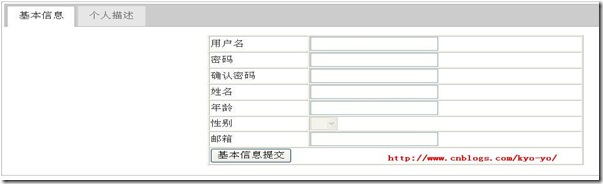#[jQuery]使用jQuery.Validate进行客户端验证（高级篇-上）——不使用微软验证控件的理由

0
0
01. 云栖社区>
2. 博客>
3. 正文

## [jQuery]使用jQuery.Validate进行客户端验证（高级篇-上）——不使用微软验证控件的理由

zting科技 2017-10-06 10:14:00 浏览624

在上一篇使用jQuery.Validate进行客户端验证（中级篇-下）中我介绍了jQuery.Validate在日常使用的过程中会遇到哪些问题及解决办法，今天的高级篇则主要是对jQuery.Validate的一些高级应用进行介绍。

1、扩展验证规则，jQuery.Validate只提供了一些基本的验证功能，并不能满足我们日常开发的需求，所以我们要为jQuery.Validate扩展验证规则。

2、分组验证，在开发的时候有时会遇到的一个问题就是，不同按钮引发不同的验证。

addMethod: function(name, method, message) {
\$.validator.methods[name] = method;
\$.validator.messages[name] = message;
if (method.length < 3) {
}
},

method-验证规则实现函数（function）

message-验证不通过显示的错误消息

jQuery.validator.addMethod("telphoneValid", function(value, element) {
var tel = /^(130|131|132|133|134|135|136|137|138|139|150|153|157|158|159|180|187|188|189)\d{8}\$/;
return tel.test(value) || this.optional(element);
}, "请输入正确的手机号码");

value：检测的对象的值

element：检测的对象1 2 3 4 5 6 7 8 9 10 11 12 13 14 15 16 17 18 19 20 21 22 23 24 25 26 27 28 `//初始化分组验证` `function InitValidationGroup() {` `    ``\$('.validationGroup .causesValidation').click(Validate);`   `    ``\$('.validationGroup :text').keydown(function(evt) {` `        ``if (evt.keyCode == 13) {` `            ``var \$nextInput = \$(this).nextAll(':input:first');`   `            ``if (\$nextInput.is(':submit')) {` `                ``Validate(evt);` `            ``}` `            ``else {` `                ``evt.preventDefault();` `                ``\$nextInput.focus();` `            ``}` `        ``}` `    ``});` `}` `function Validate(evt) {` `    ``var \$group = \$(this).parents('.validationGroup');` `    ``var isValid = true;` `    ``\$group.find(':input').each(function(i, item) {` `        ``if (!\$(item).valid())` `            ``isValid = false;` `    ``});` `    ``if (!isValid)` `        ``evt.preventDefault();` `}`

 1 2 3 4 5 6 7 8 9 10 11 12 13 14 15 16 17 18 19 20 21 22 23 24 25 26 27 28 29 30 31 32 33 34 35 36 37 38 39 40 41 42 43 44 45 46 47 48 49 50 51 52 53 54 55 56 57 58 59 60 61 62 63 64 65 66 67 68 69 70 71 72 73 74 75 76 77 78 79 80 81 82 83 84 85 86 87 `<``div` `id="tabs">` `    ``<``ul``>` `        ``<``li``><``a` `href="#baseinfo">基本信息` `        ``<``li``><``a` `href="#personaldesc">个人描述` `    ``` `    ``<``div` `id="baseinfo" class="validationGroup">` `        ``<``table` `cellpadding="1" cellspacing="1" border="1" width="50%" align="center">` `            ``<``tr``>` `                ``<``td``>` `                    ``用户名` `                ``` `                ``<``td``>` `                    ``<``asp:TextBox` `ID="txtUid" runat="server">` `                ``` `            ``` `            ``<``tr``>` `                ``<``td``>` `                    ``密码` `                ``` `                ``<``td``>` `                    ``<``asp:TextBox` `ID="txtPwd" ValidationGroup="" TextMode="Password" runat="server">` `            ``` `            ``<``tr``>` `                ``<``td``>` `                    ``确认密码` `                ``` `                ``<``td``>` `                    ``<``asp:TextBox` `ID="txtRePwd" TextMode="Password" runat="server">` `                ``` `            ``` `            ``<``tr``>` `                ``<``td``>` `                    ``姓名` `                ``` `                ``<``td``>` `                    ``<``asp:TextBox` `ID="txtName" runat="server">` `                ``` `            ``` `            ``<``tr``>` `                ``<``td``>` `                    ``年龄` `                ``` `                ``<``td``>` `                    ``<``asp:TextBox` `ID="txtAge" runat="server">` `                ``` `            ``` `            ``<``tr``>` `                ``<``td``>` `                    ``性别` `                ``` `                ``<``td``>` `                    ``<``asp:DropDownList` `ID="DropDownList1" runat="server" CssClass="required" disabled="true">` `                        ``<``asp:ListItem``>` `                        ``<``asp:ListItem` `Value="1">男` `                        ``<``asp:ListItem` `Value="0">女` `                    ``` `                ``` `            ``` `            ``<``tr``>` `                ``<``td``>` `                    ``邮箱` `                ``` `                ``<``td``>` `                    ``<``asp:TextBox` `ID="txtEmail" runat="server">` `                ``` `            ``` `            ``<``tr``>` `                ``<``td` `colspan="2">` `                    ``<``asp:Button` `ID="Button3" runat="server" Text="基本信息提交" CssClass="causesValidation"/>` `                ``` `            ``` `        ``` `    ``` `    ``<``div` `id="personaldesc" class="validationGroup">` `        ``<``p``>` `            ``<``asp:TextBox` `ID="txtDescription" runat="server" TextMode="MultiLine" Width="500px"` `                ``Height="100px">` `        ``` `        ``<``asp:Button` `ID="Button1" runat="server" Text="描述提交" CssClass="causesValidation"/>` `    ``` ``   `<``script` `type="text/javascript">` `    ``InitRules();` `    ``isValidationGroup = true;` ``

 1 2 3 4 5 6 7 8 9 10 11 12 13 14 15 16 `if (isValidationGroup) {` `    ``if (opts != undefined || opts != null) {` `        ``jQuery("#<%=form1.ClientId %>").validate(jQuery.extend(opts, { onsubmit: false }));` `    ``} else {` `        ``jQuery("#<%=form1.ClientId %>").validate({` `            ``onsubmit: false` `        ``});` `    ``}` `    ``InitValidationGroup();` `} else {` `    ``if (opts != undefined || opts != null) {` `        ``jQuery("#<%=form1.ClientId %>").validate(opts);` `    ``} else {` `        ``jQuery("#<%=form1.ClientId %>").validate();` `    ``}` `}`

PS：在jQuery.Validate进行客户端验证（高级篇-下）将为主要介绍如何使用jQuery.Validate进行ajax验证，AJAX验证的封装及简单扩展，敬请期待！

zting科技
+ 关注